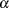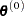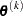The NLMIXED Procedure
 Computational Problems

### Floating-Point Errors and Overflows

Numerical optimization of a numerically integrated function is a difficult task, and the computation of the objective function and its derivatives can lead to arithmetic exceptions and overflows. A typical cause of these problems is parameters with widely varying scales. If the scaling of your parameters varies by more than a few orders of magnitude, the numerical stability of the optimization problem can be seriously reduced and can result in computational difficulties. A simple remedy is to rescale each parameter so that its final estimated value has a magnitude near 1.

If parameter rescaling does not help, consider the following actions:

• Specify the ITDETAILS option in the PROC NLMIXED statement to obtain more detailed information about when and where the problem is occurring.

• Provide different initial values or try a grid search of values.

• Use boundary constraints to avoid the region where overflows can happen.

• Delete outlying observations or subjects from the input data, if this is reasonable.

• Change the algorithm (specified in programming statements) that computes the objective function.

The line-search algorithms that work with cubic extrapolation are especially sensitive to arithmetic overflows. If an overflow occurs during a line search, you can use the INSTEP= option to reduce the length of the first trial step during the first five iterations, or you can use the DAMPSTEP or MAXSTEP option to restrict the step length of the initialin subsequent iterations. If an arithmetic overflow occurs in the first iteration of the trust region or double-dogleg algorithm, you can use the INSTEP= option to reduce the default trust region radius of the first iteration. You can also change the optimization technique or the line-search method.

### Long Run Times

PROC NLMIXED can take a long time to run for problems involving complex models, many parameters, or large input data sets. Although the optimization techniques used by PROC NLMIXED are some of the best ones available, they are not guaranteed to converge quickly for all problems. Ill-posed or misspecified models can cause the algorithms to use more extensive calculations designed to achieve convergence, and this can result in longer run times. So first make sure that your model is specified correctly, that your parameters are scaled to be of the same order of magnitude, and that your data reasonably match the model you are contemplating.

If you are using the default adaptive Gaussian quadrature algorithm and no iteration history is printing at all, then PROC NLMIXED might be bogged down trying to determine the number of quadrature points at the first set of starting values. Specifying the QPOINTS= option will bypass this stage and proceed directly to iterations; however, be aware that the likelihood approximation might not be accurate if there are too few quadrature points.

PROC NLMIXED can also have difficulty determining the number of quadrature points if the initial starting values are far from the optimum values. To obtain more accurate starting values for the model parameters, one easy method is to fit a model with no RANDOM statement. You can then use these estimates as starting values, although you will still need to specify values for the random-effects distribution. For normal-normal models, another strategy is to use METHOD=FIRO. If you can obtain estimates by using this approximate method, then they can be used as starting values for more accurate likelihood approximations.

If you are running PROC NLMIXED multiple times, you will probably want to include a statement like the following in your program:

```   ods output ParameterEstimates=pe;
```

This statement creates a SAS data set named PE upon completion of the run. In your next invocation of PROC NLMIXED, you can then specify

```   parms / data=pe;
```

to read in the previous estimates as starting values.

To speed general computations, you should double-check your programming statements to minimize the number of floating-point operations. Using auxiliary variables and factoring amenable expressions can be useful changes in this regard.

### Problems Evaluating Code for Objective Function

The starting pointmust be a point for which the programming statements can be evaluated. However, during optimization, the optimizer might iterate to a pointwhere the objective function or its derivatives cannot be evaluated. In some cases, the specification of boundary for parameters can avoid such situations. In many other cases, you can indicate that the pointis a bad point simply by returning an extremely large value for the objective function. In these cases, the optimization algorithm reduces the step length and stays closer to the point that has been evaluated successfully in the former iteration.

### No Convergence

There are a number of things to try if the optimizer fails to converge.

• Change the initial values by using a grid search specification to obtain a set of good feasible starting values.

• Change or modify the update technique or the line-search algorithm.

This method applies only to TECH=QUANEW and TECH=CONGRA. For example, if you use the default update formula and the default line-search algorithm, you can do the following:

• Change the optimization technique.

For example, if you use the default option, TECH=QUANEW, you can try one of the second-derivative methods if your problem is small or the conjugate gradient method if it is large.

The forward-difference derivatives specified with the FD= or FDHESSIAN= option might not be precise enough to satisfy strong gradient termination criteria. You might need to specify the more expensive central-difference formulas. The finite-difference intervals might be too small or too big, and the finite-difference derivatives might be erroneous.

• Double-check the data entry and program specification.

### Convergence to Stationary Point

The gradient at a stationary point is the null vector, which always leads to a zero search direction. This point satisfies the first-order termination criterion. Search directions that are based on the gradient are zero, so the algorithm terminates. There are two ways to avoid this situation:

• Use the PARMS statement to specify a grid of feasible initial points.

• Use the OPTCHECK=option to avoid terminating at the stationary point.

The signs of the eigenvalues of the (reduced) Hessian matrix contain the following information regarding a stationary point:

• If all of the eigenvalues are positive, the Hessian matrix is positive definite, and the point is a minimum point.

• If some of the eigenvalues are positive and all remaining eigenvalues are zero, the Hessian matrix is positive semidefinite, and the point is a minimum or saddle point.

• If all of the eigenvalues are negative, the Hessian matrix is negative definite, and the point is a maximum point.

• If some of the eigenvalues are negative and all remaining eigenvalues are zero, the Hessian matrix is negative semidefinite, and the point is a maximum or saddle point.

• If all of the eigenvalues are zero, the point can be a minimum, maximum, or saddle point.

### Precision of Solution

In some applications, PROC NLMIXED can result in parameter values that are not precise enough. Usually, this means that the procedure terminated at a point too far from the optimal point. The termination criteria define the size of the termination region around the optimal point. Any point inside this region can be accepted for terminating the optimization process. The default values of the termination criteria are set to satisfy a reasonable compromise between the computational effort (computer time) and the precision of the computed estimates for the most common applications. However, there are a number of circumstances in which the default values of the termination criteria specify a region that is either too large or too small.

If the termination region is too large, then it can contain points with low precision. In such cases, you should determine which termination criterion stopped the optimization process. In many applications, you can obtain a solution with higher precision simply by using the old parameter estimates as starting values in a subsequent run in which you specify a smaller value for the termination criterion that was satisfied at the former run.

If the termination region is too small, the optimization process might take longer to find a point inside such a region, or it might not even find such a point due to rounding errors in function values and derivatives. This can easily happen in applications in which finite-difference approximations of derivatives are used and the GCONV and ABSGCONV termination criteria are too small to respect rounding errors in the gradient values.Previous Page | Next Page | Top of Page